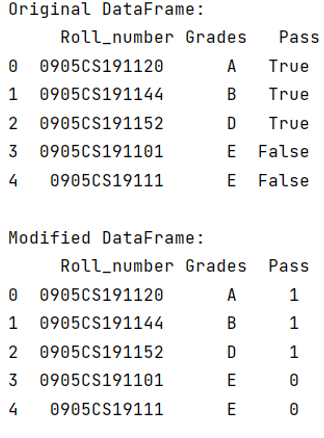# How to Map True/False to 1/0 in a Pandas DataFrame?

Given a Pandas DataFrame, we have to map True/False to 1/0.
Submitted by Pranit Sharma, on May 29, 2022

In programming, we sometimes use some specific values that an only have two values, either True or False. These values are known as Boolean values. These Boolean values have data type which has the same name i.e., Boolean. Also, Boolean values have their corresponding values with data type int also. 1 and 0 are the integer values which represent True and False respectively.

In this article, we are going to convert all the Boolean values in the format of True/False into the format of 1/0.

For this purpose, we are going to use astype() method. Let us understand with the help of an example,

To work with pandas, we need to import pandas package first, below is the syntax:

```import pandas as pd
```

Example:

```# Importing pandas package
import pandas as pd

# Creating a dictionary
d = {
'Roll_number':['0905CS191120','0905CS191144','0905CS191152','0905CS191101','0905CS19111'],
'Pass':[True,True,True,False,False]
}

# Creating a DataFrame
df = pd.DataFrame(d)

# Display original DataFrame
print("Original DataFrame:\n",df,"\n")

# Converting Boolean values True/False into 1/0
df['Pass'] = df['Pass'].astype(int)

# Display modified DataFrame
print("Modified DataFrame:\n",df)
```

Output:What's New (MCQs)

Top Interview Coding Problems/Challenges!

IncludeHelp's Blogs

Languages: » C » C++ » C++ STL » Java » Data Structure » C#.Net » Android » Kotlin » SQL
Web Technologies: » PHP » Python » JavaScript » CSS » Ajax » Node.js » Web programming/HTML
Solved programs: » C » C++ » DS » Java » C#
Aptitude que. & ans.: » C » C++ » Java » DBMS
Interview que. & ans.: » C » Embedded C » Java » SEO » HR
CS Subjects: » CS Basics » O.S. » Networks » DBMS » Embedded Systems » Cloud Computing
» Machine learning » CS Organizations » Linux » DOS
More: » Articles » Puzzles » News/Updates